16. The diagram shows a square inside an equilateral triangle. What is the value of x + y?

A:
B:
C:
D:
E:

Knave of Hearts: “I stole the tarts.”

Knave of Clubs: “The Knave of Hearts is lying."

Knave of Diamonds: “The Knave of Clubs is lying.”

Knave of Spades “The Knave of Diamonds is lying.”

How many of the four Knaves were telling the truth?

A:
B:
C:
D:

18.

What is the simplified form of the second fraction?

A: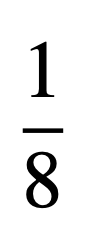B: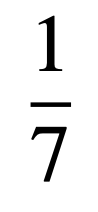C: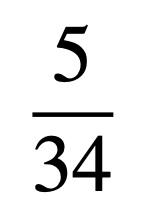D: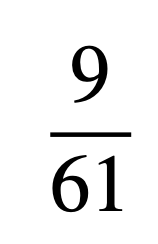E: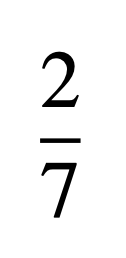19. One of the following cubes is the smallest cube that can be written as the sum of three positive cubes.

Which is it?

A:
B:
C:
D:
E:

20. The diagram shows a pyramid made up of 30 cubes, each measuring 1 m × 1 m × 1 m.

What is the total surface area of the whole pyramid (including its base)?

21. Gill is now 27 and has moved in to a new flat. She has four pictures to hang in a horizontal row on a wall which is 4800 mm wide.

The pictures are identical in size and are 420 mm wide. Gill hangs the first two pictures so that one is on the extreme left of the wall and one is on the extreme right of the wall.

She wants to hang the remaining two pictures so that all four pictures are equally spaced.

How far should Gill place the centre of each of the two remaining pictures from a vertical line down the centre of the wall?

A:B:C:D:E:22. The diagram shows a shaded region inside a regular hexagon.

The shaded region is divided into equilateral triangles.

What fraction of the area of the hexagon is shaded?

A: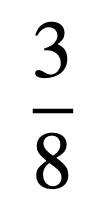B: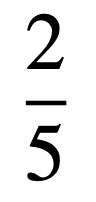C: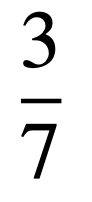D: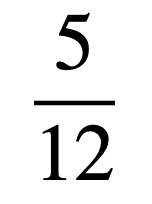E: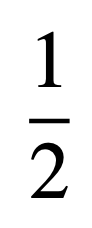23. The diagram shows four shaded glass squares, with areas of 1 cm² , 4 cm² , 9 cm²  and 16 cm² , placed in the corners of a rectangle.

The largest square overlaps two others. The area of the region inside the rectangle but not covered by any square (shown unshaded) is 1.5cm² .

What is the area of the region where the squares overlap (shown dark grey)?

A:B:C:D:E:24. A palindromic number is a number which reads the same when the order of its digits is reversed.

What is the difference between the largest and smallest five-digit palindromic numbers that are both multiples of 45?

A:
B:
C:
D:
E:

25. The four straight lines in the diagram are such that VU = VW. The sizes of ∠UXZ, ∠VYZ and ∠VZX are x°, y° and z°.

Which of the following equations gives x in terms of y and z?

A: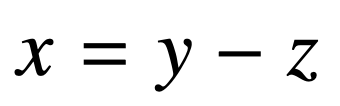B: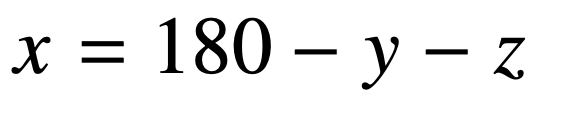C: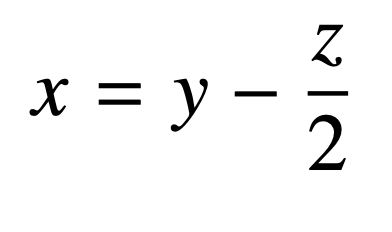D: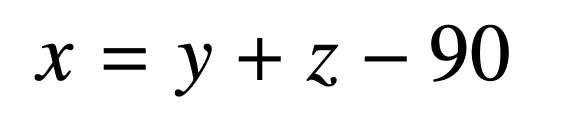E: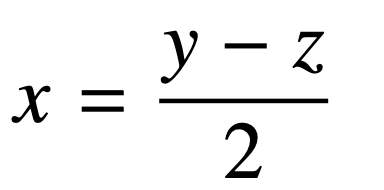Back to the top